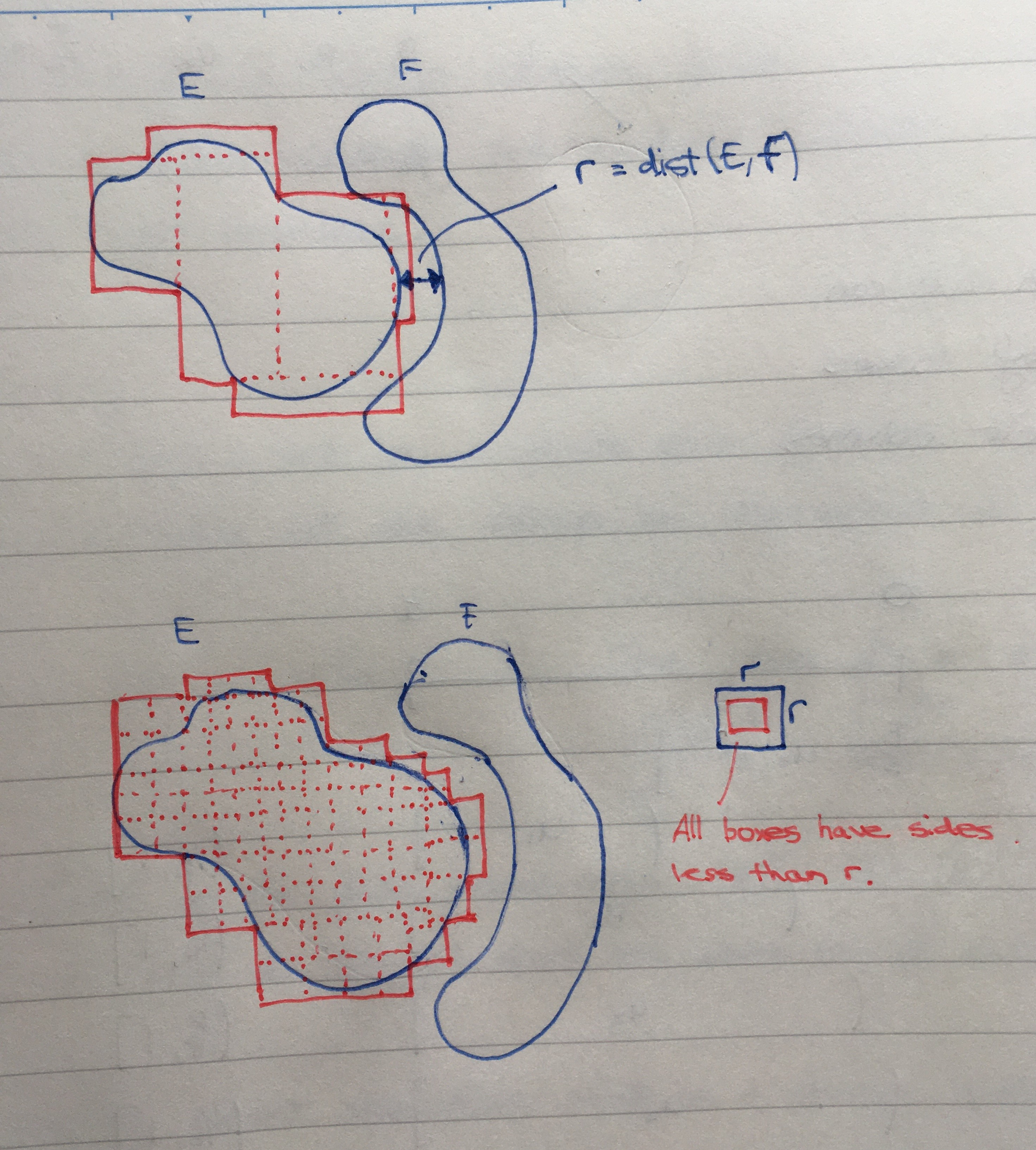Show Question
Math and science::Analysis::Tao, measure::02. Lebesgue measure

# Lebesgue outer measure. Finite additivity (for separated sets)

For Lebesgue outer measure, we left Pallet Town, so to speak, with just three properties: the measure of an empty set is zero, monotonicity and countable subadditivity. Our next step is additivity, though a restricted version of additivity: restricted to the union of just two sets, and the sets must be separated.

#### Separated sets in $$\mathbb{R}^d$$

Let $$E, F \subset \mathbb{R}^d$$ be such that $$\operatorname{dist}(E, F) > 0$$, where $$\operatorname{dist}(E, F)$$ is the distance between $$E$$ and $$F$$, defined as:

$\operatorname{dist}(E, F) := \inf(\{ |x - y| : x \in E, y \in F \} )$

When $$\operatorname{dist}(E,F) > 0$$, $$E$$ and $$F$$ are said to be separated sets.

Let $$E, F \subset \mathbb{R}^d$$ be separated sets ($$\operatorname{dist}(E, F) > 0$$). Then $$m^*(E \cup F) = m^*(E) + m^*(F)$$.

#### Essense contained within proof

Please appreciate in the following proof that no set of boxes is converted to a disjoint set of boxes. Also appreciate that $$m^*$$ is a greatest lower bound, not just any ol' real. Read something like $$m^*(E \cup F) \le m^*(E) + m^*(F)$$ as: there must be box covers of $$E \cup F$$ having a volume less-equal to the greatest lower bound required to cover $$E$$ plus the greatest lower bound required to cover $$F$$. Why? Well, we can box-cover $$E$$ with any volume less that $$m^*(E) + \varepsilon$$, and box-cover $$F$$ with any volume less than $$m^*(F) + \varepsilon$$, so we can combine these covers to cover $$E \cup F$$. In the 'worst case' with no overlapping boxes, the cover will have a volume of $$m^*E) + m^*(F) + 2 \varepsilon$$.

### Proof

Goal: for two separated sets $$E, F \subset \mathbb{R}^d$$, show that $$m^*(E \cup F) = m^*(E) + m^*(F)$$.

From countable subadditivity we have $$m^*(E \cup F) \le m^*(E) + m^*(F)$$. So we are left to show that $$m^*(E) + m^*(F) \le m^*(E \cup F)$$.

Utilize $$m^*(E \cup F)$$ to select a countable family of boxes, $$(B_n)_{n=1}^{\infty}$$ that covers $$E \cup F$$:

$\sum_{n=1}^{\infty}|B_n| \le m^*(E \cup F) + \varepsilon$

Now, make an assumption that we will later remove: suppose that each box intersects at most one of $$E$$ and $$F$$.

We can then divide the family of boxes $$(B_n)_{n=1}^{\infty}$$ into two at most countable sub-families: $$(B'_i)_{i \in I}$$ that covers $$E$$ and $$(B''_j)_{j \in J}$$ that covers $$F$$. But for $$m^*(E)$$ and $$m^*(F)$$ we have:

$m^*(E) \le \sum_{i \in I}^{}|B'_i|$

and

$m^*(F) \le \sum_{j \in J}|B''_j|$
Thus,
$m^*(E) + m^*(F) \le \sum_{i \in I}|B'_i| + \sum_{j \in J}|B''_j| = \sum_{n=1}^{\infty} |B_n|$
And in particular,
$m^*(E) + m^*(F) \le m^*(E \cup F) + \varepsilon$

#### Removing the disjoint cover assumption

The assumption that each box of the cover of $$E \cup F$$ intersects at most one of $$E$$ and $$F$$ can be removed by noting that as $$\operatorname{dist}(E, F) = r > 0$$, all boxes in a cover of $$E \cup F$$ can be partitioned such that the diameter of each box is less than $$r$$, and in this case, none of the smaller boxes will intersect both $$E \cup F$$.#### Jordan outer measure finite additivity

The argument above applies regardless of whether the box cover is finite or countable, so it could be used for the finite additivity for Jordan outer measure too. Jordan outer measure already has finite additivity established, but only for when the sets involved are Jordan measurable. The Jordan measurability allowed us to infer the finite additivity of the Jordan outer measure simply by equating it to the inner measure, for which the finite additivity is easily proved due to any inner cover necessarily being disjoint.

### Example

#### Disjoint closed sets, with one being compact ⟹ dist > 0

Let $$E, F \subset \mathbb{R}^d$$. If $$E$$ and $$F$$ are disjoint closed sets, and at least one of them is compact, then $$\operatorname{dist}(E, F) > 0$$. In this case, we have finite additivity for Lebesgue outer measure.

Rough proof. If $$\operatorname{dist}(E, F) = 0$$, then we could create a sequence in the compact (and thus bounded) set which converges to an element of the other set. But sequences in a closed bounded set must converge within the set. So we would have an element that belongs to both sets, which is a contradiction.

p19